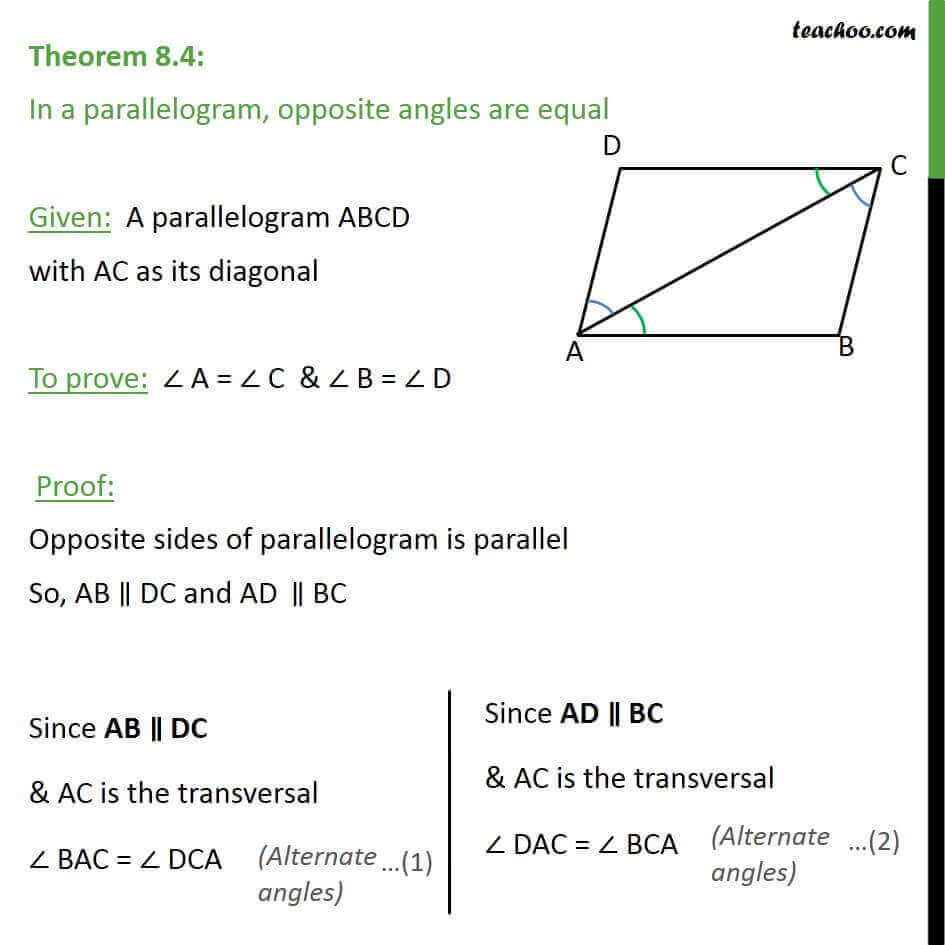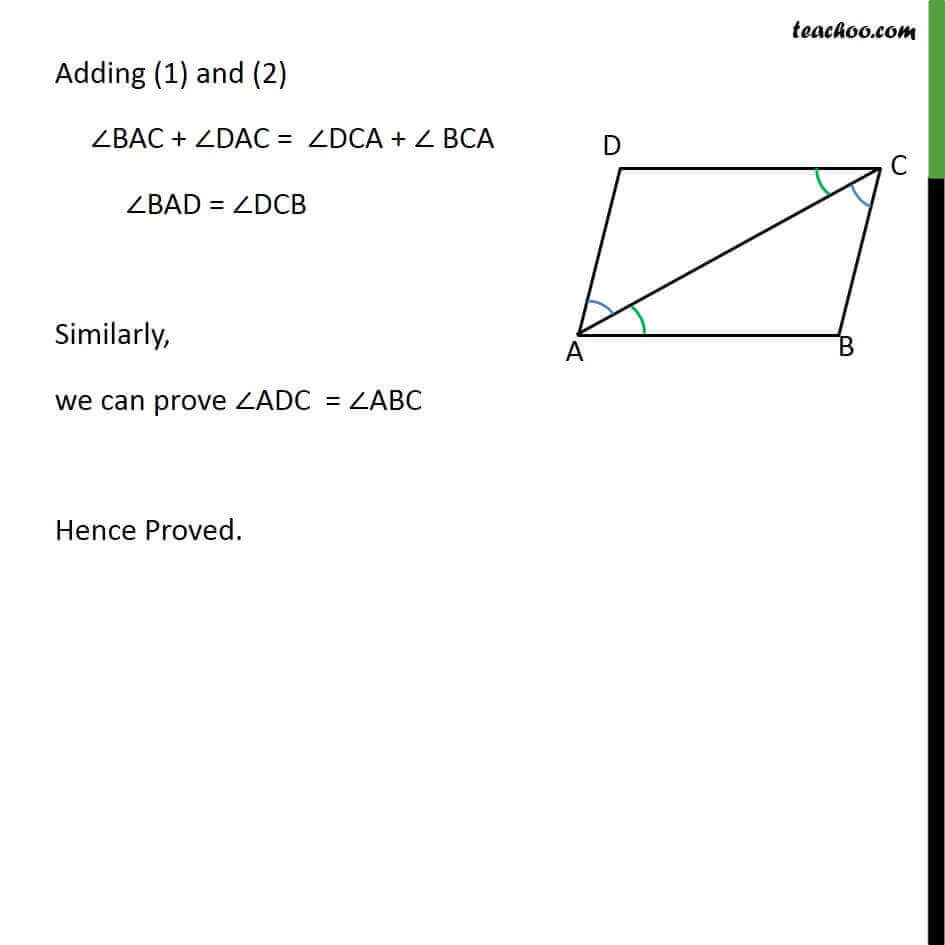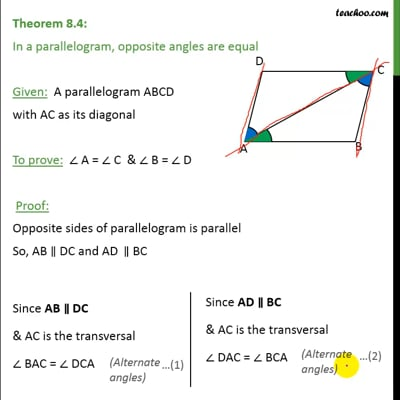Theorems

Chapter 8 Class 9 Quadrilaterals (Term 2)
Serial order wiseThis video is only available for Teachoo black users

### Transcript

Theorem 8.4: In a parallelogram, opposite angles are equal Given: A parallelogram ABCD with AC as its diagonal To prove: ∠ A = ∠ C & ∠ B = ∠ D Proof: Opposite sides of parallelogram is parallel So, AB ∥ DC and AD‖∥ BC Adding (1) and (2) ∠BAC + ∠DAC = ∠DCA + ∠ BCA ∠BAD = ∠DCB Similarly, we can prove ∠ADC = ∠ABC Hence Proved.